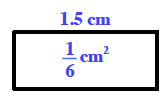### Home > CC1MN > Chapter 8 > Lesson 8.2.1 > Problem8-70

8-70.

A rectangle has an area of $\frac { 1 } { 6 }$ square centimeters and a length of $1.5$ centimeters. What is the width? What is the perimeter?

Here is a diagram of the given information to help you find the perimeter.
You will need to find the width first.